Documentation

## Waveform Analysis Using the Ambiguity Function

This example illustrates how to use the ambiguity function to analyze waveforms. It compares the range and Doppler capability of several basic waveforms, e.g., the rectangular waveform and the linear and stepped FM waveform.

In a radar system, the choice of a radar waveform plays an important role in enabling the system to separate two closely located targets, in either range or speed. Therefore, it is often necessary to examine a waveform and understand its resolution and ambiguity in both range and speed domains. In radar, the range is measured using the delay and the speed is measured using the Doppler shift. Thus, the range and the speed are used interchangeably with the delay and the Doppler.

### Introduction

To improve the signal to noise ratio (SNR), modern radar systems often employ the matched filter in the receiver chain. The ambiguity function of a waveform represents exactly the output of the matched filter when the specified waveform is used as the filter input. This exact representation makes the ambiguity function a popular tool for designing and analyzing waveforms. This approach provides the insight of the resolution capability in both delay and Doppler domains for a given waveform. Based on this analysis, one can then determine whether a waveform is suitable for a particular application.

The following sections use the ambiguity function to explore the range-Doppler relationship for several popular waveforms. To establish a comparison baseline, assume that the design specification of the radar system requires a maximum unambiguous range of 15 km and a range resolution of 1.5 km. For the sake of simplicity, also use 3e8 m/s as the speed of light.

Rmax = 15e3; Rres = 1500; c = 3e8;

Based on the design specifications already mentioned, the pulse repetition frequency (PRF) and the bandwidth of the waveform can be computed as follows.

prf = c/(2*Rmax); bw = c/(2*Rres);

Choose a sampling frequency that is twice of the bandwidth.

fs = 2*bw;

### Rectangular Pulse Waveform

The simplest waveform for a radar system is probably a rectangular waveform, sometimes also referred to as single frequency waveform. For the rectangular waveform, the pulse width is the reciprocal of the bandwidth.

A rectangular waveform can be created as follows.

rectwaveform = phased.RectangularWaveform('SampleRate',fs,... 'PRF',prf,'PulseWidth',1/bw)
rectwaveform = phased.RectangularWaveform with properties: SampleRate: 200000 DurationSpecification: 'Pulse width' PulseWidth: 1.0000e-05 PRF: 10000 PRFSelectionInputPort: false OutputFormat: 'Pulses' NumPulses: 1 PRFOutputPort: false 

Because the analysis of a waveform is always performed on full pulses, keep the OutputFormat property as 'Pulses'. One can also check the bandwidth of the waveform using the bandwidth method.

bw_rect = bandwidth(rectwaveform)
bw_rect = 1.0000e+05 

The resulting bandwidth matches the requirement. Now, generate one pulse of the waveform, and then examine it using the ambiguity function.

wav = rectwaveform(); ambgfun(wav,rectwaveform.SampleRate,rectwaveform.PRF);In the figure, notice that the nonzero response is occupying only about 10% of all delays, focusing in a narrow strip around delay 0. This occurs because the waveform has a duty cycle of 0.1.

dc_rect = dutycycle(rectwaveform.PulseWidth,rectwaveform.PRF)
dc_rect = 0.1000 

When investigating a waveform's resolution capability, the zero delay cut and the zero Doppler cut of the waveform ambiguity function are often of interest.

The zero Doppler cut of the ambiguity function returns the auto-correlation function (ACF) of the rectangular waveform. The cut can be plotted using the following command.

ambgfun(wav,rectwaveform.SampleRate,rectwaveform.PRF,'Cut','Doppler');The zero Doppler cut of the ambiguity function depicts the matched filter response of a target when the target is stationary. From the plot, one can see that the first null response appears at 10 microseconds, which means that this waveform could resolve two targets that are at least 10 microseconds, or 1.5 km apart. Hence, the response matches the requirement in the design specification.

The zero delay cut can be plotted using similar syntax.

ambgfun(wav,rectwaveform.SampleRate,rectwaveform.PRF,'Cut','Delay');Notice that the returned zero delay response is fairly broad. The first null does not appear till at the edge, which corresponds to a Doppler shift of 100 kHz. Thus, if the two targets are at the same range, they need to have a difference of 100 kHz in the Doppler domain to be separated. Assuming the radar is working at 1 GHz, according to the computation below, such a separation corresponds to a speed difference of 30 km/s. Because this number is so large, essentially one cannot separate two targets in the Doppler domain using this system.

fc = 1e9; deltav_rect = dop2speed(100e3,c/fc)
deltav_rect = 30000 

At this point it may be worth to mention another issue with the rectangular waveform. For a rectangular waveform, the range resolution is determined by the pulse width. Thus, to achieve good range resolution, the system needs to adopt very small pulse width. At the same time, the system also needs to be able to send out enough energy to the space so that the returned echo can be reliably detected. Hence, a narrow pulse width requires very high peak power at the transmitter. In practice, producing such power can be very costly.

### Linear FM Pulse Waveform

One can see from the previous section that the Doppler resolution for a single rectangular pulse is fairly poor. In fact, the Doppler resolution for a single rectangular pulse is given by the reciprocal of its pulse width. Recall that the delay resolution of a rectangular waveform is given by its pulse width. Apparently, there exists a conflict of interest between range and Doppler resolutions of a rectangular waveform.

The root issue here is that both the delay and the Doppler resolution depend on the pulse width in opposite ways. Therefore, one way to solve this issue is to come up a waveform that decouples this dependency. One can then improve the resolution in both domains simultaneously.

Linear FM waveform is just such a waveform. The range resolution of a linear FM waveform is no longer depending on the pulse width. Instead, the range resolution is determined by the sweep bandwidth.

In linear FM waveform, because the range resolution is now determined by the sweep bandwidth, the system can afford a longer pulse width. Hence, the power requirement is alleviated. Meanwhile, because of the longer pulse width, the Doppler resolution improves. This improvement occurs even though the Doppler resolution of a linear FM waveform is still given by the reciprocal of the pulse width.

Now, explore the linear FM waveform in detail. The linear FM waveform that provides the desired range resolution can be constructed as follows.

lfmwaveform = phased.LinearFMWaveform('SampleRate',fs,... 'SweepBandwidth',bw,'PRF',prf,'PulseWidth',5/bw)
lfmwaveform = phased.LinearFMWaveform with properties: SampleRate: 200000 DurationSpecification: 'Pulse width' PulseWidth: 5.0000e-05 PRF: 10000 PRFSelectionInputPort: false SweepBandwidth: 100000 SweepDirection: 'Up' SweepInterval: 'Positive' Envelope: 'Rectangular' OutputFormat: 'Pulses' NumPulses: 1 PRFOutputPort: false 

The pulse width is 5 times longer than that of the rectangular waveform used in the earlier sections of this example. Notice that the bandwidth of the linear FM waveform is the same as the rectangular waveform.

bw_lfm = bandwidth(lfmwaveform)
bw_lfm = 100000 

The zero Doppler cut of the linear FM waveform appears in the next plot.

wav = lfmwaveform(); ambgfun(wav,lfmwaveform.SampleRate,lfmwaveform.PRF,'Cut','Doppler');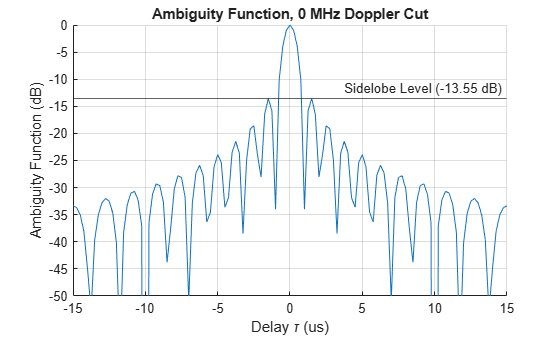From the preceding figure, one can see that even though the response now has sidelobes, the first null still appears at 10 microseconds, so the range resolution is preserved.

One can also plot the zero delay cut of the linear FM waveform. Observe that the first null in Doppler domain is now at around 20 kHz, which is 1/5 of the original rectangular waveform.

ambgfun(wav,lfmwaveform.SampleRate,lfmwaveform.PRF,'Cut','Delay');Following the same procedure as for the rectangular waveform in the earlier sections of this example, one can calculate that the 20 kHz Doppler separation translates to a speed difference of 6 km/s. This resolution is 5 times better than the rectangular waveform. Unfortunately, such resolution is still inadequate.

deltav_lfm = dop2speed(20e3,c/fc)
deltav_lfm = 6000 

One may also be interested in seeing the 3-D plot of the ambiguity function for the linear FM waveform. If you want to see a 3-D plot other than the contour format, you can just get the returned ambiguity function and then plot is using your favorite format. For example, the following snippet generates the surface plot of the linear FM waveform ambiguity function.

[afmag_lfm,delay_lfm,doppler_lfm] = ambgfun(wav,lfmwaveform.SampleRate,... lfmwaveform.PRF); surf(delay_lfm*1e6,doppler_lfm/1e3,afmag_lfm,'LineStyle','none'); axis tight; grid on; view([140,35]); colorbar; xlabel('Delay \tau (us)');ylabel('Doppler f_d (kHz)'); title('Linear FM Pulse Waveform Ambiguity Function');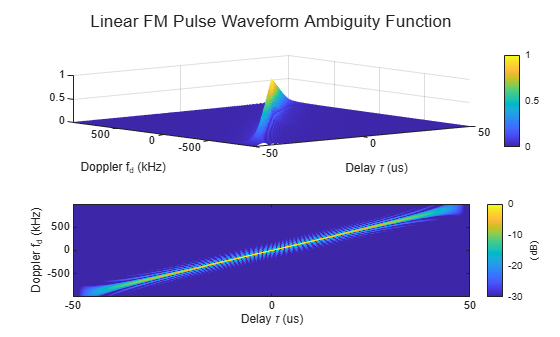Notice that compared to the ambiguity function of the rectangular waveform, the ambiguity function of the linear FM waveform is slightly tilted. The tilt provides the improved resolution in the zero delay cut. The ambiguity function of both rectangular waveform and linear FM waveform have the shape of a long, narrow edge. This kind of ambiguity function is often termed as "knife edge" ambiguity function.

Before proceeding to improve further the Doppler resolution, it is worth looking at an important figure of merit used in waveform analysis. The product of the pulse width and the bandwidth of a waveform is called the waveform's time bandwidth product. For a rectangular waveform, the time bandwidth product is always 1. For a linear FM waveform, because of the decoupling of the bandwidth and the pulse width, the time bandwidth can be larger than 1. The waveform just used has a time bandwidth product of 5. Recall that by preserving the same range resolution as the rectangular waveform, the linear FM waveform achieves a Doppler resolution that is 5 times better.

### Coherent Pulse Train

As of the previous section, the Doppler resolution of the linear FM waveform is still fairly poor. One way to improve this resolution is to further extend the pulse width. However, this approach will not work for two reasons:

• The duty cycle of the waveform is already 50%, which is close to the practical limit. (Even if one could, say, use a 100% duty cycle, it is still only a factor of 2 improvement, which is far from being able to resolve the issue.)

• Longer pulse width means large minimum detectable range, which is also undesirable.

If one cannot extend the pulse width within one pulse, one has to look beyond this boundary. Indeed, in modern radar systems, the Doppler processing often uses a coherent pulse train. The more pulses in the pulse train, the finer the Doppler resolution.

To illustrate the idea, next, try a five-pulse burst.

release(lfmwaveform); lfmwaveform.NumPulses = 5; wav = lfmwaveform();

First, plot the zero Doppler cut of the ambiguity function.

ambgfun(wav,lfmwaveform.SampleRate,lfmwaveform.PRF,'Cut','Doppler');Notice that for the zero Doppler cut, the first null is still around 10 microseconds, so the range resolution is the same. One should see immediately the presence of many range domain sidelobes. These sidelobes are the tradeoff for using a pulse train. The distance between the mainlobe and the first sidelobe is the length of one entire pulse, i.e., the reciprocal of the PRF. As one can see, this value corresponds to the maximum unambiguous range.

T_max = 1/prf
T_max = 1.0000e-04 

The zero delay cut also has sidelobes because of the pulse train. The distance between the mainlobe and the first sidelobe is the PRF. Thus, this value is the maximum unambiguous Doppler the radar system can detect. One can also calculate the corresponding maximum unambiguous speed.

ambgfun(wav,lfmwaveform.SampleRate,lfmwaveform.PRF,'Cut','Delay');V_max = dop2speed(lfmwaveform.PRF,c/fc)
V_max = 3000 

However, notice that the mainlobe is now much sharper. Careful examination reveals that the first null is at about 2 kHz. This Doppler resolution can actually be obtained by the following equation,

deltaf_train = lfmwaveform.PRF/5
deltaf_train = 2000 

i.e., the resolution is now determined by the length of our entire pulse train, not the pulse width of a single pulse. The corresponding speed resolution is now

deltav_train = dop2speed(deltaf_train,c/fc)
deltav_train = 600 

which is significantly better. More importantly, to get even finer speed resolution, one can simply increase the number of pulses included in the pulse train. Of course the number of pulses one can have in a burst depends on whether one can preserve the coherence for the entire duration, but that discussion is out of the scope of this example.

One may notice that in the zero delay cut, the distance between the peaks are no longer constant, especially for farther out sidelobes. This lack of constancy occurs because the linear FM waveform's ambiguity function is tilted. Hence, judging the separation of sidelobes in zero delay cut can be misleading. The ambiguity caused by the pulse train is probably best viewed in the contoured form, as the next code example shows. Notice that along the edge of the ambiguity function, those sidelobes are indeed evenly spaced.

ambgfun(wav,lfmwaveform.SampleRate,lfmwaveform.PRF);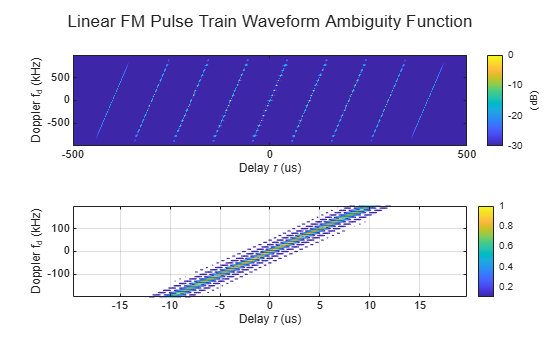Because of all the sidelobes, this kind of ambiguity function is called bed of nails ambiguity function.

### Stepped FM Waveform

The linear FM waveform is very widely used in radar systems. However, it does present some challenges to the hardware. For one thing, the hardware has to be able to sweep the entire frequency range in one pulse. Using this waveform also makes it harder to build the receiver because it has to accommodate the entire bandwidth.

To avoid these issues, you can use a stepped FM waveform instead. A stepped FM waveform consists of multiple contiguous CW pulses. Each pulse has a different frequency and together, all pulses occupy the entire bandwidth. Hence, there is no more sweep within the pulse, and the receiver only needs to accommodate the bandwidth that is the reciprocal of the pulse width of a single pulse.

Next, set up such a stepped FM waveform.

stepfmwaveform = phased.SteppedFMWaveform('SampleRate', fs,... 'PulseWidth',5/bw,'PRF',prf,'NumSteps',5,'FrequencyStep',bw/5,... 'NumPulses',5)
stepfmwaveform = phased.SteppedFMWaveform with properties: SampleRate: 200000 DurationSpecification: 'Pulse width' PulseWidth: 5.0000e-05 PRF: 10000 PRFSelectionInputPort: false FrequencyStep: 20000 NumSteps: 5 OutputFormat: 'Pulses' NumPulses: 5 PRFOutputPort: false 
wav = stepfmwaveform();

The zero Doppler cut, zero delay cut, and contour plot of the ambiguity function are shown below.

ambgfun(wav,stepfmwaveform.SampleRate,stepfmwaveform.PRF,'Cut','Doppler');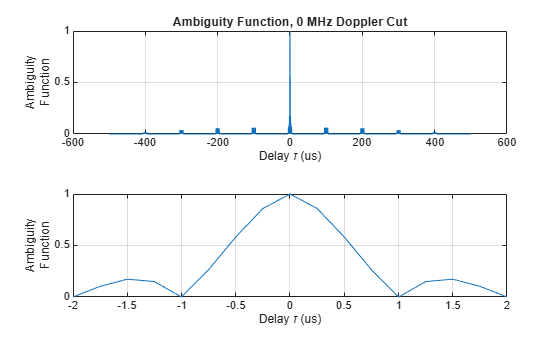ambgfun(wav,stepfmwaveform.SampleRate,stepfmwaveform.PRF,'Cut','Delay');ambgfun(wav,stepfmwaveform.SampleRate,stepfmwaveform.PRF);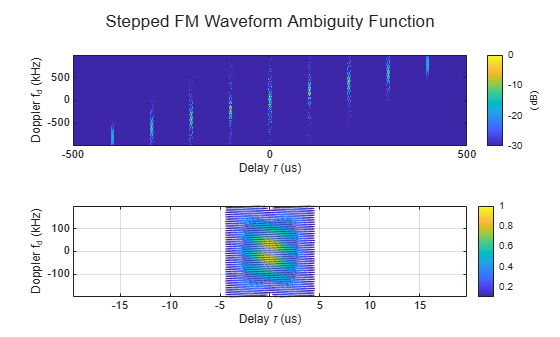From these figures, one can make the following observations:

• The first null in delay is still at 10 microseconds, so the range resolution is preserved. Notice that because each pulse is different, the sidelobes in the range domain disappear.

• The first null in Doppler is still at 2 kHz, so it has the same Doppler resolution as the 5-pulse linear FM pulse train. The sidelobes in the Doppler domain still present as in the linear FM pulse train case.

• The contour plot of the stepped FM waveform is also of type bed of nails. Although the unambiguous range is greatly extended, the unambiguous Doppler is still confined by the waveform's PRF.

As to the disadvantage of a stepped FM waveform, the processing becomes more complicated.

### Barker-Coded Waveform

Another important group of waveforms is phase-coded waveforms, among which the popularly used ones are Barker codes, Frank codes, and Zadoff-Chu codes. In a phase-coded waveform, a pulse is divided into multiple subpulses, often referred to as chips, and each chip is modulated with a given phase. All phase-coded waveforms have good autocorrelation properties which make them good candidates for pulse compression. Thus, if a phase-coded waveform is adopted, it could lower the probability of interception as the energy is spread into chips. At the receiver, a properly configured matched filter could suppress the noise and achieve good range resolution.

Barker code is probably the most well known phase-coded waveform. A Barker-coded waveform can be constructed using the following command.

barkerwaveform = phased.PhaseCodedWaveform('Code','Barker','NumChips',7,... 'SampleRate', fs,'ChipWidth',1/bw,'PRF',prf)
barkerwaveform = phased.PhaseCodedWaveform with properties: SampleRate: 200000 Code: 'Barker' ChipWidth: 1.0000e-05 NumChips: 7 PRF: 10000 PRFSelectionInputPort: false OutputFormat: 'Pulses' NumPulses: 1 PRFOutputPort: false 
wav = barkerwaveform();

This Barker code consists of 7 chips. Its zero Doppler cut of the ambiguity function is given by

ambgfun(wav,barkerwaveform.SampleRate,barkerwaveform.PRF,'Cut','Doppler');From the figure, one can see that the zero Doppler cut of a Barker code's ambiguity function has an interesting property. All its sidelobes have the same height and are exactly 1/7 of the mainlobe. In fact, a length-N Barker code can provide a peak-to-peak suppression of N, which helps distinguish closely located targets in range. This is the most important property of the Barker code. The range resolution is approximately 10 microseconds, the same as the chip width.

There are two issues associated with a Barker code. First, there are only seven known Barker codes. Their lengths are 2, 3, 4, 5, 7, 11 and 13. It is believed that there are no other Barker codes. Second, the Doppler performance of the Barker code is fairly poor. Although the ambiguity function has good shape at the zero Doppler cut, once there is some Doppler shift, the sidelobe level increases significantly. The increase can be seen in the following contour plot.

ambgfun(wav,barkerwaveform.SampleRate,barkerwaveform.PRF);### Summary

This example compared several popular waveforms including the rectangular waveform, the linear FM waveform, the stepped FM waveform and the Barker-coded waveform. It also showed how to use the ambiguity function to analyze these waveforms and determine their resolution capabilities.